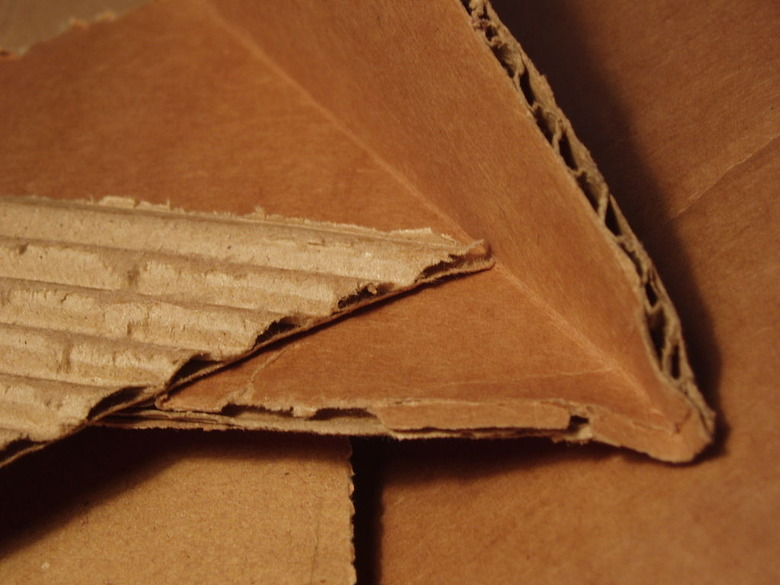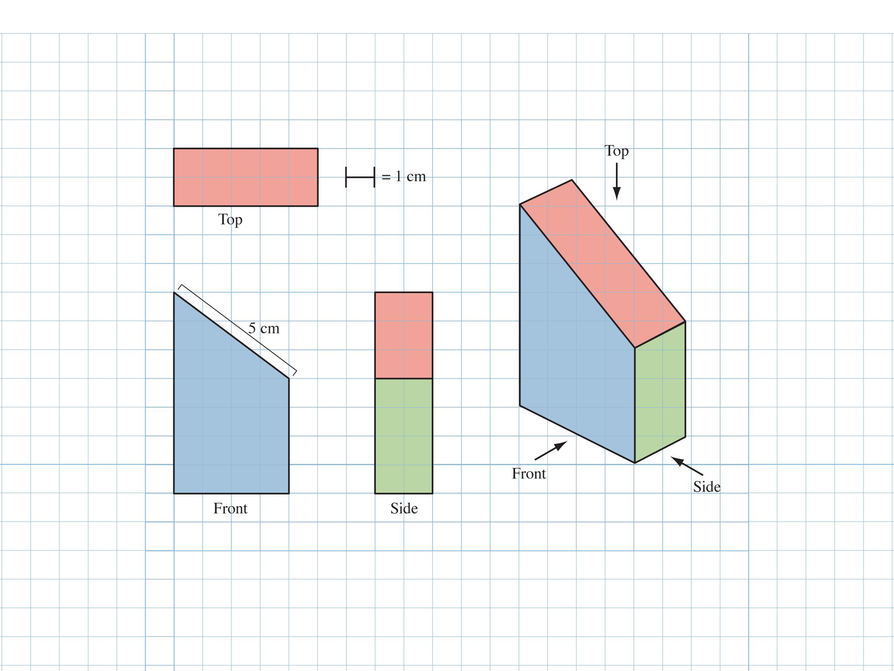Subject:
Geometry
Material Type:
Lesson Plan
Level:
Middle School
7
Provider:
Pearson
Tags:
7th Grade Mathematics, Models, Prisms, Scale, Surface Area, Volume
Language:
English
Media Formats:
Text/HTML

# Unit Review# Lesson Overview

Students will work on the final portion of their project which includes creating the nets for the sides, making a slice in one of their buildings, and putting their buildings together. Once their two model buildings are complete, they will find the surface area and volume for their models and the full-size buildings their models represent.

# Key Concepts

The second part of the project is essentially a review of the second half of the unit, while still using scale drawings. Students will find the surface area of a prism as well as the surface area of a truncated prism. The second prism will require estimating and problem solving to figure out the net and find the surface area. Students will also be drawing the figure using scale to find actual surface area.

# Goals

• Redraw a scale drawing at a different scale.
• Find measurements using a scale drawing.
• Find the surface area of a prism.

SWD: Students with disabilities may have a more challenging time identifying areas of improvement to target in their projects. It may be helpful to model explicitly for students (using an example project or student sample) how to review a project using the rubric to assess and plan for revisions based on that assessment.

Students with fine motor difficulties may require grid paper with a larger scale. Whenever motor tasks are required, consider adaptive tools or supplementary materials that may benefit students with disabilities.

Students with disabilities may struggle to recall prerequisite skills as they move through the project. It may be necessary to check in with students to review and reinforce estimation skills.

# Lesson Guide

Have students view the project rubric. Give students 2 minutes to study the rubric. Then have students take turns saying one thing about the rubric without looking at it. When students are finished, tell them that today they will add any specifics to the rubric that they think are needed for evaluating their projects.

Have 1-inch grid paper ready for students, as well as the base sheets. Students should also have access to rulers and protractors.

# Review Project Rubric

Today you will continue to work on your project with your partner.

• Before you start, review the project rubric.

# Lesson Guide

Discuss the Math Mission. Students will work on their project and evaluate their progress using the project rubric.

ELL: This lesson offers students a rich opportunity to use academic vocabulary. Before engaging in the discussion, consider allowing ELLs, when possible, to discuss in their primary language with other ELLs who share the same primary language.

## Opening

Work on your project and evaluate your progress using the project rubric.

# Lesson Guide

Students will spend the majority of the time working on the project.

Allow time at the end of the period for the Self Check.

# Preparing for Ways of Thinking

Look for students who demonstrate the following strategies to be shared during the class discussion:

• Students who worked out methods to find the net of the sliced prism
• Students who worked out methods to find the surface area of the sliced prism
• Students who decided to alter their design to make it easier to make the net and find its surface area
• Students who quickly realized the edges of the lateral surfaces will not all fall on grid lines and will need to be measured to match the base sides

# Mathematical Practices

Mathematical Practice 1: Make Sense of Problems and Persevere in Solving Them

Look for students who successfully build the sliced prism and have a good explanation of how they found the surface area.

Mathematical Practice 3: Construct Viable Arguments and Critique the Reasoning of Others

Look for students who understand how to find the actual surface area and can explain it clearly. As students work, look for students who can justify their thinking.

Mathematical Practice 5: Use Appropriate Tools Strategically

Some students will realize that the accuracy of their drawing will be enhanced by using the grid and at the same time realize that the grid will not work for all edges.

Mathematical Practice 6: Attend to Precision

Look for students who use the correct units in their calculations.

# Project Work

Today, you and your partner should try to complete the following steps.

• Sketch a scale version of the nets for your model buildings using the inch grid. Remember, your scale is 20 ft to 1 in. The net for the sliced prism will be tricky to draw and will require some guesswork with regard to finding the proper angles and lengths. Think about ways to construct the slice.
• Using your scale drawings as guides, draw the actual nets for each building on 1-inch grid paper. Cut out each net.
• Construct each model. Adjust the cut on the sliced building if you need to.
• Mount the models on their bases.
• Find the surface area of the full-size buildings and the model buildings. Show your work and justify each step. Note that your calculations (particularly for the sliced surface) will be estimates rather than exact measures.
• Find the volume of the full-size buildings and the model buildings. Show your work and justify each step.

## Hint:

• As you work, use the project rubric to evaluate your progress and make sure you are on the right track.

# Mathematics

Discuss the work students completed today. Ask questions such as these:

• How did you decide what the net should look like?
• How did you find the surface area of the prism?
• How did you find the actual surface area?
• What strategies did you use to find the surface area of the sliced prism?
• How did [student names] organize their thoughts differently? Which way of thinking makes more sense to you? Which way of thinking brought out the structure of the mathematics?
• How did [student names] make sense of the problem?
• Could you state what [student names] said in a different way?
• How could you measure more accurately?

ELL: As with other discussions, consider presenting some of the questions in writing to support ELLs. Further, consider providing sentence frames, such as the following (in the order the questions were given):

If the questions are shown in writing, students may not need to have sentence frames at this point.

• I decided what the net should look like by…
• I found the surface of the area of the prism by…

# Ways of Thinking: Make Connections

Take notes about your classmates’ successes and failures to gain insight into ways that you might improve your project.

# Lesson Guide

This task allows you to assess students’ work and determine what difficulties they are having. The results of the Self Check will help you determine which students should work on the Gallery and which students would benefit from review before the assessment. Have students work on the Self Check individually.

# Assessment

Have students submit their work to you. Make notes on what their work reveals about their current levels of understanding and their different problem-solving approaches.

Do not score students’ work. Share with each student the most appropriate Interventions to guide their thought process. Also, note students with a particular issue so that you can work with them in the Putting It Together lesson that follows.

SWD: Students with disabilities may benefit from having the Self Check presented in a variety of ways (e.g., as auditory and visual information). Provide students the option of listening to the content for this task. Some students with disabilities may need additional time to complete the Self Check. Be sure to make provisions for the additional time or consider reducing the number of tasks required for students to demonstrate mastery of skill.

ELL: When reviewing work done by ELLs, make sure that a poor use of the language is not interpreted as a poor understanding of the topic.

# Interventions

Student does not calculate the surface area correctly.

• Can you break up the front surface into figures you know the area of?
• What are the length and width of each side?
• How many sides are there?

Student does not calculate the volume correctly.

• What would the figure look like if you turned it?
• Can you break up the front surface into figures you know the volume of?
• How thick is the figure?

Student provides a poor explanation.

For example: The student explains calculations rather than giving mathematical reasons.

• How did you find the surface area/volume?
• Why did you use those numbers?

Student provides adequate solution to all questions.

• Find a different way of solving the problem.

1. The rectangle is 2 cm wide and 5 cm long. It appears to be 4 cm long in the top view because the figure is 4 cm long, and this is how the surface would appear.

2. The surface area is 84 cm${}^{2}$. There are 6 surfaces to account for. The front/back can be broken into a square and a right triangle:

Front/Back: (4 cm ⋅ 4 cm) + (4 cm ⋅ 3 cm)${}^{2}$ = 16 cm${}^{2}$ + 6 cm${}^{2}$ = 22 cm${}^{2}$
22 cm${}^{2}$ ⋅ 2 = 44 cm${}^{2}$

Top: 5 cm ⋅ 2 cm = 10 cm${}^{2}$

Bottom: 4 cm ⋅ 2 cm = 8 cm${}^{2}$

Side: 2 cm ⋅ 4 cm = 8 cm${}^{2}$

Opposite Side: 2 cm ⋅ 7 cm = 14 cm${}^{2}$

Total: 44 cm${}^{2}$ + 10 cm${}^{2}$ + 8 cm${}^{2}$ + 8 cm${}^{2}$ + 14 cm${}^{2}$ = 84 cm${}^{2}$

Alternately, students could look at the figure as a trapezoidal prism and calculate the area of the bases plus the lateral surface area:

Base: 22 cm${}^{2}$

Lateral Surface Area: (5 cm + 4 cm + 4 cm + 7 cm)(2 cm) = (20 cm ⋅ 2 cm) = 40 cm${}^{2}$

Total: 2(22 cm${}^{2}$) + 40 cm${}^{2}$ = 44 cm${}^{2}$ + 40 cm${}^{2}$ = 84 cm${}^{2}$

3. The volume is 44 cm${}^{3}$. If the trapezoid is considered the base (whose area is 22 cm${}^{2}$), then that area can be multiplied by the “height” (2 cm):

22 cm${}^{2}$ ⋅ 2 cm = 44 cm${}^{3}$

The figure could also be broken up into two parts—a rectangular prism and a half-rectangular prism: (4 cm ⋅ 4 cm ⋅ 2 cm) + (4 cm ⋅ 3 cm ⋅ 2 cm${}^{2}$) = 32 cm${}^{3}$ + 12 cm${}^{3}$ = 44 cm${}^{3}$

# Self Check: Find Surface Area and Volume

Complete this Self Check by yourself.

Use the drawings to answer the following questions. Note that the surface areas of the top and bottom surfaces will not be the same because the prism has been sliced. For the same reason, the surface areas of the side surfaces will be different.

1. What is the length and width of the rectangle cross section revealed?
2. What is the surface area of the figure? Show how you found your answer.
3. What is the volume of the figure? Show how you found your answer.# Lesson Guide

Have each student write a brief reflection before the end of class. Review the reflections to see students’ work about their project. If you notice insightful comments, plan to share them with the class in the next lesson.

ELL: Allow some additional time for ELLs to discuss with a partner before writing, to help them organize their thoughts. Allow ELLs who share the same primary language to discuss in their language of choice, if they wish, and to use a dictionary.

# Reflection

Write a reflection about the ideas discussed in class today. Use the sentence starter below if you find it to be helpful.

One thing about our project that my partner and I need to improve on before our presentation is …